# Rectangular field

One dimension of the rectangular field is 56 m greater than second dimension. If each side of the rectangle increases by 10 m, increases the surface field is 1480 m2 . Determine dimencions of the field.

a =  41 m
b =  97 m

### Step-by-step explanation:Did you find an error or inaccuracy? Feel free to write us. Thank you!Tips to related online calculators
Do you have a system of equations and looking for calculator system of linear equations?

#### You need to know the following knowledge to solve this word math problem:

We encourage you to watch this tutorial video on this math problem:

## Related math problems and questions:

• The hallThe hall had a rectangular ground plan one dimension 20 m longer than the other. After rebuilding the length of the hall declined by 5 m and the width has increased by 10 m. Floor area increased by 300 m2. What were the original dimensions of the hall?
• Rectangles - sidesOne side of the rectangle is 10 cm longer than a second. Shortens longer side by 6 cm and extends shorter by 14 cm increases the rectangle area by 130 cm2. What are the dimensions of the original rectangle?
• Rectangular field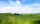What is the area of a rectangular field 100m by 8m?
• SeedsThe field has a rectangular shape with dimensions of 128 m and 350 m. How many kg of seed are needed for sowing if the 1 m2 will consume 25 g of seeds?
• Two lands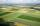The common area of the two neighboring lands is 964 m2. The second land is 77 m2 smaller than twice the size of the first land. Find the areas of each land.
• Square vs rectangleSquare and rectangle have the same area contents. The length of the rectangle is 9 greater and width 6 less than side of the square. Calculate the side of a square.
• Rectangular landDue to the new road, the longer dimension of the rectangular plot had to be shortened by 4 percent, and the shorter dimension shrunk by 10 percent. By how many percent has the area of land decreased?
• GardensThe garden has the square shape with circumference 124 m. Divide it into two rectangular gardens, one should circumference 10 meters more than the second. What size will have a gardens?
• Area and perimeter of rectangleThe content area of the rectangle is 3000 cm2, one dimension is 10 cm larger than the other. Determine the perimeter of the rectangle.
• The fieldThe player crossed the field diagonally and walked the length of 250 m. Calculate the length of the field, circumference if one side of field 25 meters.
• Land areas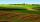Two land areas are 244 m2. The first parcel is 40 m2 less than twice the second one. What has the acreage of each parcel?
• Square sides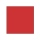If the square side increases by 28%, the square circumference increases by 11.2 meters. Find the length of the original square side.
• Extending square gardenMrs. Petrová's garden had the shape of a square with a side length of 15 m. After its enlargement by 64 m2 (square), it had the shape of a square again. How many meters has the length of each side of the garden been extended?
• Rectangle vs squareOne side of the rectangle is 1 cm shorter than the side of the square, the second side is 3 cm longer than the side of the square. Square and rectangle have the same content. Calculate the length of the sides of a square and a rectangle.
• Two gardens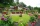The total area of the two gardens is 864 m2. The first garden is 60 m2 smaller than three times the second garden. What is the area of each garden?
• Pool tilesThe pool is 25m long, 10m wide, and 160cm deep. How many m2 of tiles will be needed on the walls and the pool? How many tiles are needed when 1 tile has a square shape with a 20cm side? How much does it cost when 1m2 of tiles costs 258 Kc?
• The rectangleThe rectangle has a circumference of 32 m. One side is 2 m longer than the other side. Calculate the side lengths of this rectangle.